# Sequences

What is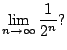You may have encountered sequences long ago in earlier courses and they seemed very difficult. You know much more mathematics now, so they will probably seem easier. On the other hand, we're going to go very quickly.

 We will completely skip several topics from Chapter 11. I will try to make what we skip clear. Note that the homework has been modified to reflect the omitted topics.

A sequence is an ordered list of numbers. These numbers may be real, complex, etc., etc., but in this book we will focus entirely on sequences of real numbers. For example,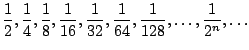Since the sequence is ordered, we can view it as a function with domain the natural numbers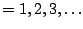.

Definition 6.1.1 (Sequence)   A sequence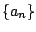is a function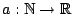that takes a natural number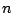to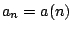. The number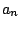is theth term.

For example,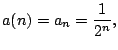which we write as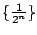. Here's another example: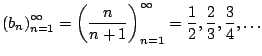Example 6.1.2   The Fibonacci sequence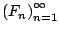is defined recursively as follows: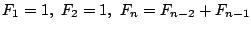for $n &ge#geq;3$Let's return to the sequence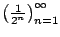. We write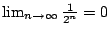, since the terms get arbitrarily small.

Definition 6.1.3 (Limit of sequence)   If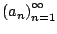is a sequence then that sequence converges to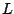, written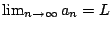, ifgets arbitrarily close toasget sufficiently large. SECRET RIGOROUS DEFINITION: For every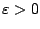there exists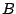such that for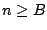we have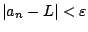.

This is exactly like what we did in the previous course when we considered limits of functions. If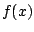is a function, the meaning of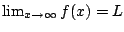is essentially the same. In fact, we have the following fact.

Proposition 6.1.4   If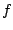is a function withandis the sequence given by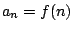, then.

As a corollary, note that this implies that all the facts about limits that you know from functions also apply to sequences!

Example 6.1.5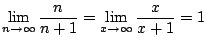Example 6.1.6   The converse of Proposition 6.1.4 is false in general, i.e., knowing the limit of the sequence converges doesn't imply that the limit of the function converges. We have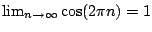, but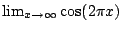diverges. The converse is OK if the limit involving the function converges.

Example 6.1.7   Compute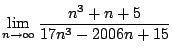. Answer: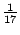.

William Stein 2006-03-15